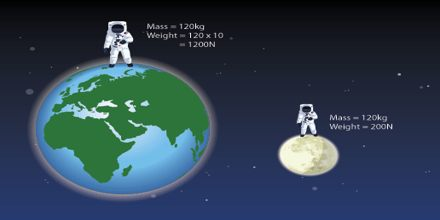Physics

# What are Mass and Weight?Prime objective of this lecture is to present on Mass and Weight. Mass is the amount of matter in an object and is measured in kilograms. It is a scientific measure of the amount of matter an object is made up of. No matter where you are at given moment in time, mass is constant. Weight is a form of measurement that is dependent on gravity and, unlike mass, your weight can vary depending on where you are in the universe. Weight has different values depending on where the object is in the Universe. In a sentence mass is a measurement of how much matter is in an object; weight is a measurement of how hard gravity is pulling on that object.